Mobile QR Code1. (Research Institute (Attached), ASTERASYS Co., Ltd., Seoul, Korea skw123@asterasys.co.kr)
2. (Research Institute (Attached), AirLab, Gimhae, Korea bcyoo79@gmail.com)
3. (School of Biomedical Engineering, Inje University, Korea hhchoi@inje.ac.kr )

HIFU, Microbubble, Simulation, Thermal effect

## 1. Introduction

Ultrasound is one of the most widely used diagnostics in the medical imaging field because it is non-invasive and generally less expensive than other imaging techniques. It also has developed into a very successful modality in clinical diagnosis because it can provide real-time images of soft tissue structures and blood flow. However, it does not have sharp contrast and is sometimes distorted due to its own artifacts. But with the development of ultrasound contrast agents (UCAs), including microbubbles, this problem can be partially resolved.

The interaction of microbubbles with ultrasound has been studied in the last 30 years, and it is broadly used in diagnostic medical applications. As a UCA, microbubbles consist of 1 to 10-μm encapsulated gas-filled bubbles. One of their major characteristics is that they oscillate strongly under an external ultrasound field. The backscattering echo intensity is proportional to the change in acoustic impedance between the blood and microbubbles and acts as an echo-enhancer in diagnostic ultrasound. Another aspect that is impressive is the possibility of using microbubbles to carry various drugs to a target region and rupturing them by localized ultrasound energy. There are numerous results that show that they may also have therapeutic applications as drug or gene-delivery agents and as a mechanism for bypassing the blood-brain barrier [1,2].

Therapeutic ultrasound was considered as a tool for hyperthermia or thermal ablation of tumors. Ultrasound acoustic power induces destruction and collapse of a microbubble, produces a high-amplitude response, and can produce strong microstreaming. The local heat deposition is amplified when microbubbles are present as cavitation or microbubble-enhanced heating .

High-intensity focused ultrasound (HIFU) is a therapeutic medical technology where high-pressure acoustic radiation is focused on an Region Of Interest (ROI) within the body to heat tissue. HIFU has gained interest because it is non-invasive and has potential to treat cancers, such as those in the liver and brain [4,5]. But HIFU causes unwanted tissue damage during the treatment with long-treatment time and damages normal tissue before the target region. To reduce damage to target tissues, resting time has to be increased while modulating the intensity level .

Cavitation or microbubble-enhanced heating effects can be reduced by the acoustic intensity or treatment time required for the HIFU effect. Since the early 2000s, research to artificially induce the thermal enhancement effect by microbubbles and use it for HIFU treatment has been in progress. Kajiyama studied the optimal ultrasound pulse in a heating characteristic experiment that used HIFU after injecting a UCA . Yu evaluated HIFU treatment using a UCA through the tissue necrosis rate depending on whether the UCA was injected into a rabbit liver .

Recently, as the potential for the efficacy and safety of HIFU enhancement using microbubbles has begun to draw attention, clinical trials for actual patients have been increasing. Wang Jingqi clinically tested 102 patients with adenomyosis. When HIFU treatment was performed after injection of ultrasound contrast medium, it was confirmed that it was safer than the result of treatment with only HIFU with less energy and reduced average power . Cheng conducted clinical trials with 63 rabbits and 143 patients with uterine fibroids. As a result, it was confirmed that the group injected with UCA was able to treat fibroids in a much shorter time than the group without UCA, and there were no major complications . However, most of the studies to date are limited to the research results through the experience that UCA can cause the thermal enhancement effect of HIFU. In order to be used clinically in practice, a quantitative analysis of microbubbles and a quantitative study under conditions close to reality are required.

Gnanaskandan numerically analyzed the reinforcement effect of microbubbles using the Keller-Herring bubble dynamic equation and compared the simulation results with actual experimental results using the microbubble distribution model. However, the shape is different from a group of microbubbles actually formed by modeling microbubbles in a cube-shaped cylinder . Kamei analyzed the non-linear HIFU enhancement effect by microbubbles, but there is still insufficient experimental content to verify the theory .

Therefore, we present a method for quantitatively analyzing and predicting the heat enhancement effect by a microbubble cloud collected during HIFU irradiation. To this end, the behavior of microbubbles was mathematically analyzed, and a simulation was performed by modeling a group of microbubbles, in which are collected form similar to the actual fluids field. In order to verify the simulation, an experiment was performed under the same conditions as the simulation.

The paper is organized as follows. In Section 2, we introduce the background knowledge on a microbubble and its behavior equations and theories used in this study, such as the Rayleigh-Plesset equation, modified Herring model, and bio-heat transfer equation (BHTE). Section 3 describes a simulation program using the proposed microbubble behavior-analyzing method for quantitatively analyzing and predicting the heat effect. In section 4, the simulation condition and experimental variables are introduced. In Section 5, we describe the compared microbubble behavior results of the simulation and experiment for the heating effect. Finally, Section 6 explains the conclusions of this study and describes the limitations.

## 2. Background

In this section, theoretical studies are introduced to describe a microbubble that as a UCA. Also, we discuss a bio-heat transfer equation for ultrasound acoustic energy transfer to thermal energy. These discussed background studies are applied for the microbubble-cloud heating-effect simulation and experimental tests.

### 2.1 Microbubble used as UCA

A microbubble UCA consists of a phospholipid coating film, which has surface tension and viscoelasticity. Because a phospholipid film suppresses vibrations caused by ultrasound irradiation, it acts as a basic variable in the behavior analysis. Surface tension ($\sigma$) is measured as a force per unit length, and the definition can be expressed by Eq. (1).

##### (1)
σ = d y n c m = 1 e r g c m 2 = 1 10 7 m N 10 4 m 2

The shear viscosity changes due to the continuously changing shear speed during the expansion of the gas bubble. The shear speed can be explained by Eq. (2), and the shear viscosity is defined by Eq. (3).

##### (2)
r ˙ i = p i m i + r y i x ^ ,    p ˙ i = F i γ p y i x ^

##### (3)
η =   V k T 0 d t p x y   0 p x y t

where $p_{xy}\left(t\right)$ is the non-equilibrium average of the pressure tensor using the dynamic equation of motion.

### 2.2 Microbubble behavior Equation

Since 1917, several theoretical models have been introduced to study gas bubble dynamics in liquids, and early study on the geometric analysis of microbubble behavior began with Rayleigh's work, which focused on the application of fluid mechanics . Later, Plesset conceived a mathematical model called the Rayleigh-Plesset equation based on Rayleigh's study . This is the most commonly used formula to describe a single microbubble vibration. But these models are the simplest models because a microbubble is driven by just a low-amplitude sound field in an infinite fluid. This model ignores the liquid compressibility effects and assumes that the gas pressure in a microbubble is uniform and follows the polytropic law. The Rayleigh-Plesset equation is shown in Eq. (4):

##### (4)
ρ R ¨ R + 3 2 R ˙ 2 = p g t p 0 p i t 4 η R ˙ R 2 σ R

where $R_{0}$ is the microbubble radius at equilibrium, $\dot{R}$ and $\ddot{R}$ represent the first- and second-order derivatives of the microbubble radius $R$, $p_{0}$ is the hydrostatic pressure, $p_{i}\left(t\right)$ is the incident ultrasound pressure in the liquid at an infinite distance, $p_{g}\left(t\right)$ is the uniform gas pressure within the microbubble, and $\rho$, $\sigma$, and $\eta$ are the density of surrounding liquid, surface tension of the microbubble-liquid interface, and viscosity of the bulk fluid, respectively.

In general, UCA is a form in which a film constrains a gas inside a bubble, and characteristics such as the thickness and viscosity of the film must be considered. De Jong and Hoff modeled encapsulated microbubbles by incorporating experimentally determined elastic and friction parameters into the Rayleigh-Plesset model. They studied it based on the Herring model, which considered the changes of pressure for liquid compressibility modified by Morgan [14,15]. The modified Herring model is shown in Eq. (5):

##### (5)
ρ R R ¨ + 3 2 ρ R ˙ 2 = p 0 + 2 σ R 0 + 2 χ R 0 R 0 R 3 γ 1 3 γ c R ˙ 12 μ s h ε R ˙ R R ε p 0 + P t

where $\rho$ is the density of the surrounding liquid (assumed to be constant), $R$, $\dot{R}$, and $\ddot{R}$ are the radius, change in velocity, and acceleration of the microbubble, respectively, and $R_{0}$ is the initial radius. $p_{0}$ is the atmospheric pressure, and $P\left(t\right)$ is the external pressure (that is, the pressure by HIFU). $\sigma$ is the surface tension, $\chi$ is the elasticity of the microbubble shell, $\gamma$ is the polytropic index, $\mu$ is the viscosity of the medium, $\mu _{sh}$ is the viscosity of the microbubble shell, and $\varepsilon$ is the thickness of the shell.

Through Eq. (5), the behavior according to the external pressure applied to a microbubble can be analyzed. The behavior of microbubbles generates additional acoustic pressure, and the pressure can be obtained from the pressure equation according to the distance defined in the Rayleigh-Plesset model. Eq. (6) shows the pressure equation according to distance:

##### (6)
P r a d r , r b , t = ρ R r b 2 R ˙ 2 + R R ¨

where $r_{b}$ represents the distance away from the center of the microbubble.

### 2.3 Bio-heat Transfer Equation

Ultrasound acoustic power-induced destruction and collapse of a microbubble produces high microbubble-enhanced heating in vasculature and tissue. The complex thermal interaction between the vasculature and tissue has been a topic of interest for many researchers. Harry H. Pennes presented the first quantitative relationship describing heat transfer in human tissue including the effects of blood flow on tissue temperature on a continuum basis . For small volumes, the rate of heat generation is calculated by the attenuation coefficient in the tissue and the time-average intensity of the ultrasound.

The transfer of heat takes place through mechanisms such as conduction, convection, radiation, metabolism, evaporation, and phase change. In consideration of this, Pennes proposed a heat transfer model called the Bio-Heat Transfer Equation (BHTE), as shown in Eq. (7):

##### (7)
ρ C T t = · k T W b C b T T a + Q

where $\rho$ is tissue density, $C$ and $C_{b}$ are the specific heats of tissue and blood, $W_{b}$ is the perfusion rate of blood, $T_{a}$ is the temperature of blood vessels, $k$ is the thermal conductivity of tissue, and $Q$ is the rate of heat production by ultrasound destruction and collapse. In this study, we developed a modified BHTE to understand the thermal effect propagation caused by microbubbles oscillation and explosion, which is given by Eq. (8):

##### (8)
ρ C T t = k T W b C b T T a + Q H i f u + Q B u b b l e

where $Q_{Hifu}$ and $Q_{\textit{Bubble}}$ are the thermal effects induced by HIFU irradiation and microbubbles behaviors, respectively.

## 3. Simulation Program

The thermal effect caused by the interaction between a microbubble cloud and the HIFU was simulated using a 3-D numerical model or structural model. This section is organized as follows. We first present a microbubble cloud behavior model that considers the microbubble's distribution in a cloud volume. Then, we introduce a program used to calculate the thermal effect of interaction between a developed microbubble cloud and the microbubble behavior model.

### 3.1 Microbubble Cloud Modeling

The UCA does not exist as a single microbubble but exists in the form of a large number of microbubbles collected in an aqueous solution, so the clustered microbubble cloud should be considered. In addition, the distance of each microbubble is a very important variable for calculating the delivery pressure of the microbubble cloud, so it is important to set the cloud shape and the distance between the microbubbles in modeling the microbubble cloud. We first considered a cube-array bubble cloud suggested by Tatsuya Moriyama et al. . The cube-array microbubble cloud method has the advantage of being simple and intuitive for modeling because all microbubbles are located at equal intervals. However, the distance between the microbubbles is very diverse because the focal point of the HIFU located in the center is very narrow and small. This is a major cause of slowing down the simulation processing speed.

Therefore, in this study, a spherical array method involving spherical microbubble clouds with multiple layers of shells was chosen. The microbubbles on each shell have the same distance to the target. This means that the microbubbles on the same shell transmit the same pressure to the target, so the computation speed of the simulation can be improved. Fig. 1 illustrates the spherical microbubble cloud model.

Fig. 1. Proposed microbubble cloud model: (a) diagram; (b) 3D simulation results.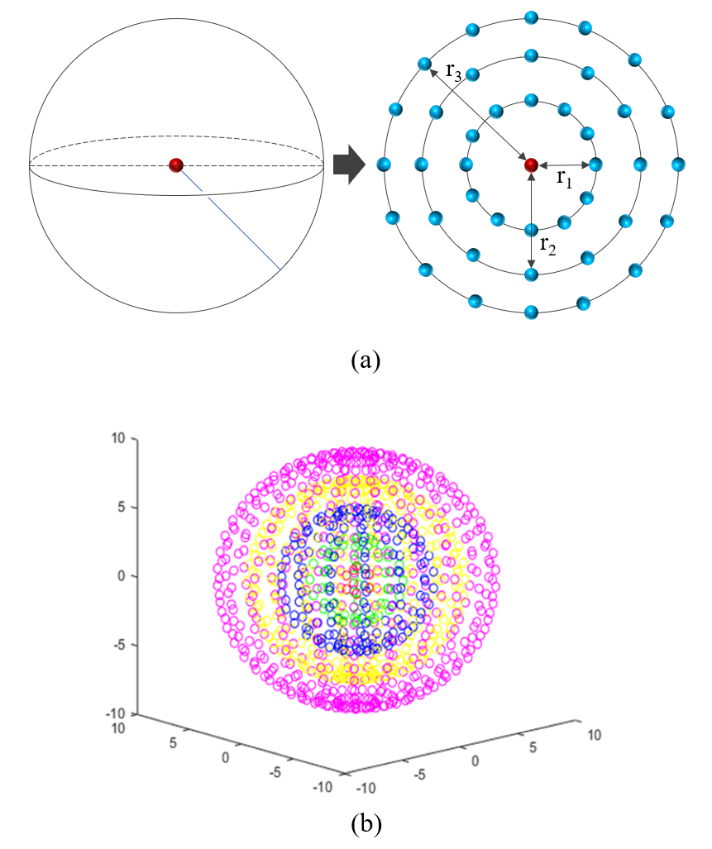### 3.2 Simulation Program Algorithm

Fig. 2 shows the produced simulation program. The simulation presented in this paper was designed as follows. First, in order to analyze the behavior of microbubbles, the modified Herring model was solved using a 6$^{\mathrm{th}}$-order Runge-Kutta numerical analysis. Second, single microbubbles were placed on the modeled microbubble group model, and the distance to the target was calculated to obtain the total pressure applied to the target. Finally, the total pressure applied to the target was converted to thermal energy through BHTE, and then the expected temperature was calculated.

Fig. 2. Developed simulation program UI and simulation parameters.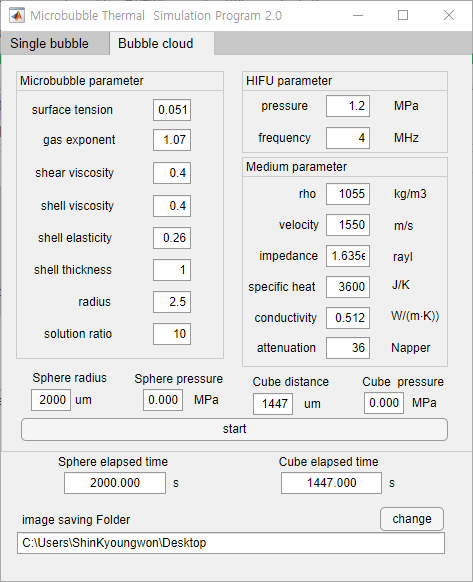MATLAB 2018b was used for all simulation procedures. This simulation program was designed to control the characteristics of the microbubbles, the concentration of the microbubble cloud, the size of the microbubble cloud, the characteristics of the medium, and the HIFU characteristics. It was also set to display a graph of the temperature rise over time.

However, this simulation program and procedure have some assumptions:

· First, all microbubbles are completely spherical and all have the same characteristics.

· Second, all microbubbles are evenly spread in the microbubble group.

· Third, the explosion of microbubbles all occurs within one cycle of HIFU ultrasound.

· Finally, the interaction between microbubbles is ignored.

## 4. Outline of the Experiment

In order to investigate the ability of UCA to increase the thermal effect of HIFU, several systems were assembled, and an experimental procedure was established.

### 4.1 The Contrast Agent

To verify the UCA ability for the thermal effect, the commercially available SonoVue$^{\mathrm{TM}}$ was selected. It is a novel ultrasound contrast medium made of phospho-lipid-stabilized microbubbles of sulfur hexafluoride (SF$_{6}$) and a poorly soluble and totally innocuous gas. It presents outstanding stability and resistance to pressure. SonoVue$^{\mathrm{TM}}$ appears as a white, milky solution and is stable for hours.

Because no sterilizing agent is present in the composition, SonoVue$^{\mathrm{TM}}$ should be used within 6 hours after reconstitution. SonoVue$^{\mathrm{TM}}$ is isotonic, and its viscosity is similar to that of blood. It does not contain protein-based materials (Table 1).

Table 1. SonoVue$^{\mathrm{TM}}$ Characteristics.

 SonoVueTM Parameter Characteristic Bubble diameter 2.5 [μm] Bubble volume concentration ~ 5 [μL/mL] Surface tension σ 0.051 [N/m] Polytropic gas exponent γ 1.07 [N/m] Shell elasticity χ 0.26 [N/m] Shell viscosity μ s h 0 to 8 [N/m] Shell thickness ε $1\times 10^{-9}$ [m]

Table 2. Acoustic parameter of phantom and human. tissue

 Parameter NIPAM Soft tissue Polyolefin tube Vessel Sound velocity $\left[\mathrm{m}/\mathrm{s}\right]$ 1,505 1,540 1,515 1,513 Attenuation $\left[\mathrm{dB}/\mathrm{cm}\right]$ 0.50 0.70 1.18 1.45 Density [$\mathrm{kg}/m^{3}]$ 999.32 1.080.00 0.92 1.07 Impedance [$\text{Mrayl}]$ 1.520 1.620 1.394 1.560

### 4.2 Ultrasound Phantom Parameters

A phantom was fabricated using N-isopropylacrylamide (NIPAM), which is similar to soft tissue and has acoustic characteristics of the human body. We also used polyolefin tubes, which have similar characteristics to blood vessels and were implanted to inject the ultrasonic contrast agent (Fig. 3). In addition, in order to evaluate the temperature rise, a thermocouple intersected with the tube, and a liquid mixed with a blood-mimicking material filled the tube. Table 2 compares the acoustic parameters of human tissue and tissue-mimicking phantom materials

### 4.3 Experiment Setup

To evaluate the thermal effect ability by UCA in HIFU therapy, an experimental system was set up as shown in Fig. 4. The phantom system ($30\times 30\times 30mm$$^{3}$) includes a polyolefin tube ($d=5mm$) for use in microbubble capture and a thermocouple implanted orthogonally to the polyolefin tube for measurement of temperature changes. The temperature changes were continuously monitored by Datalogger (Graphtec, Japan) from the implanted thermocouple. A set of a commercial focused ultrasound surgical units (ASTERASYS, Korea) that includes a HIFU transducer was used during the experiment. The HIFU transducer produces a peak acoustic intensity of $1.2MPa$ at the main lobe. The frequency was set to $4MHz$. For focusing point adjustment, a transducer was attached to a 3-axis jig system. The initial temperature of the blood-like substance mixed with microbubbles and the phantom was set to 25$^{\circ}$C

### 4.4 Experiment Procedure

Prior to the main experiments, to evaluate the proposed spherical microbubble cloud model and simulation program, an experiment and comparison were conducted by changing the mixing ratio of the UCA and the blood-mimicking material from 1:4 to 1:1. The main experiment consists of measuring the change in the thermal effect according to the volume and the concentration of the microbubble cloud. First, a temperature increase was simulated by increasing the radius of the microbubble cloud from 0.5 mm to 1.5 mm at 0.25-mm intervals using a 30% concentration of UCA. Second, using a microbubble cloud with a radius of 1 mm, the temperature increase was simulated by increasing the concentration of UCA from 10% to 50% in 10% increments. Fig. 5 shows a diagram of the experimental procedure, and Table 3 shows parameters for the simulation study.

## 5. Evaluation Results

### 5.1 Spherical Microbubble Cloud Model

To evaluate the spherical microbubble cloud model and simulation program, an actual experiment and comparison were conducted. Table 4 shows the simulation results, and Table 5 shows the results of HIFU irradiation experiments using an ultrasonic phantom. In the absence of microbubbles, the simulation results and experimental results increased by 5.12$^{\circ}$C and 5.1$^{\circ}$C to 30.12$^{\circ}$C and 30.1$^{\circ}$C, respectively. After that, in the case of mixed contrast agent, it was confirmed that both the simulation and experiment showed additional heat increase. Also, the heat-enhancing effect increased as the proportion of microbubbles increased. At a 1:1 ratio, a temperature increase of 6.92$^{\circ}$C was confirmed through simulation, and a temperature increase of 7$^{\circ}$C was confirmed in the ultrasonic phantom.

Fig. 6. Simulation and experiment results of the maximum temperature according to the ratio of solution.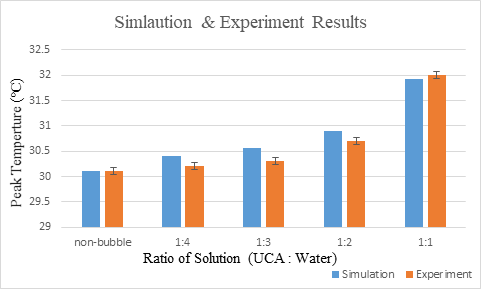Table 3. Simulation parameter for UCA thermal effect.

 Simulation No. Microbubble-cloud radius [mm] UCA solution ratio [%] 1 0.5 30 0.75 1.0 1.25 1.5 2 1.0 10 20 30 40 50

Table 4. Temperature rise simulation result.

 Simulation Result Unit [℃] Non-bubble 1:4 1:3 1:2 1:1 Init temp 25 Peak temp 30.12 30.40 30.56 30.89 31.92 Delta T 5.12 5.40 5.56 5.89 6.92

Table 5. Temperature rise experiment result.

 Experiment Result Unit [℃] Non-bubble 1:4 1:3 1:2 1:1 Init temp 25 Peak temp 30.1 30.2 30.3 30.76 32 Delta T 5.1 5.2 5.3 5.7 7

Fig. 6 shows a graph of the simulation results according to the concentration of the ultrasonic contrast agent and the temperature rise result of the experiment using the ultrasonic phantom. Simulation results and experimental results using ultrasonic phantoms show that the temperature rise according to each condition has error within 0.2, and the temperature rise pattern is similar. This means that the analysis of the thermal enhancement effect through quantitative analysis of the microbubble cloud presented in this study is significant.

### 5.2 Thermal Effect of HIFU according to the Characteristics of Microbubble Cloud

The thermal enhancement effect of HIFU according to the characteristics of the microbubble cloud was evaluated through simulation using the simulation program. The factors that have the greatest influence on the thermal enhancement effect of HIFU are the number of microbubbles in the medium and the distance between the focal point and the microbubbles. This is closely related to the size of the microbubble cloud and the concentration of the ultrasonic contrast medium. Therefore, in this study, the temperature increase was confirmed and evaluated through simulation while changing the volume of the microbubble cloud and the concentration of the ultrasonic contrast medium. Fig. 7 shows a simulation result of the temperature rise with no microbubbles.

### 5.3 Thermal Effect according to the Volume of Microbubble Cloud

While changing the diameter of the microbubble cloud, the medium was fixed at 30%, and the microbubble group radius was increased from 0.5 mm to 1.5 mm. When the radius of the microbubble group was 0.5 mm, it increased by 0.1025$^{\circ}$C compared to when the microbubbles were not present. After that, it was confirmed that the temperature exponentially increased as the size of the microbubble cloud increased.

In Section 3.2, microbubbles separated by more than 25 μm were found to have little effect. It is considered that the increase in the total pressure due to the increase in the number of microbubbles and the increase in the size of the microbubble cloud has more effect than the attenuation due to the distance. Table 6 and Fig. 8 show the heat rise results with increasing radius of the microbubble cloud.

### 5.4 Thermal Effect according to UCA Solution Ratio

The radius of the microbubble cloud was set to 1 mm, and the temperature increase was confirmed through a simulation while increasing the collection density of the microbubbles (that is, the concentration of the ultrasonic contrast agent). The thermal enhancement effect of the microbubble group increased from 10% to 50% according to the increase in the concentration of the ultrasonic contrast agent. This confirmed that an additional exponential temperature increase occurred. When the ultrasonic focusing point and the microbubbles become closer, a large amount of microbubbles can be collected in a group of microbubbles of the same size, thereby inducing a synergistic heat effect. Table 7 and Fig. 9 show the results of thermal rise according to the microbubble concentration.

Table 6. Simulation results according to microbubble-cloud volume.

 UCA Solution Ratio [%] Microbubble cloud radius [mm] Peak Temperature [°C] 30 0.5 29.29 0.75 29.3687 1.0 29.7257 1.25 30.5072 1.5 31.8956

Table 7. Simulation results according to microbubble-cloud concentration ratio.

 Microbubble cloud radius [mm] UCA Solution Ratio [%] Peak Temperature [°C] 1 10 29.2507 20 29.4282 30 29.7257 40 30.1286 50 30.7017

Fig. 7. Temperature simulation result without microbubble cloud.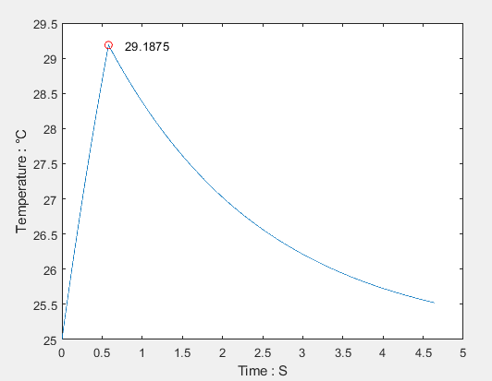Fig. 8. Simulation results according to microbubble-cloud volume.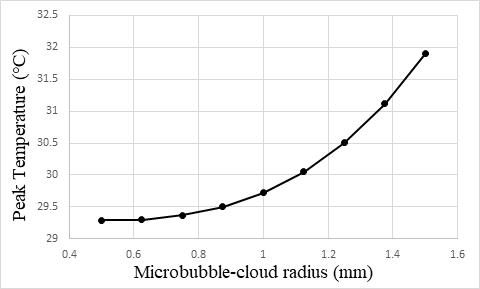Fig. 9. Results of thermal rise according to the microbubble concentration.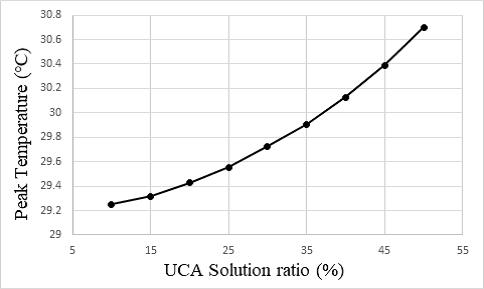## 6. Conclusion

This study confirmed the effect of the characteristics of a microbubble cloud on the thermal enhancement during HIFU irradiation and analyzed it quantitatively. To do this, a microbubble behavior model was mathematically analyzed, and a microbubble group was modeled to simulate the microbubble distribution in ultrasonic contrast medium. After that, a simulation program to check the thermal enhancement effect was made using the microbubble behavior model and the microbubble group model, and the effect of the characteristics of the microbubble group on the thermal enhancement effect of HIFU was confirmed.

The microbubble behavior of ultrasonic contrast medium was simulated using the modified Herring model. At this time, since the energy change according to the sound speed was limited, the change in the sound speed due to the HIFU pressure was not considered in the simulation. In addition, in the actual experiment, it was expected that there would be limitations in the collection of microbubbles and the temperature rise due to ultrasonic physical phenomena such as scattering. Therefore, it was expected that the accuracy of the temperature rise result could be improved if the conditions of the temperature saturation point are confirmed through additional experiments and applied to the simulation program.

In addition, in the simulations produced by this study, all microbubbles had the same properties as the microbubbles spread out in the shell of the spherical microbubble cloud model. Practical results can be obtained by applying an algorithm that randomly applies the arrangement and characteristics of microbubbles to the extent physically possible. The results of this study are expected to contribute to the development of HIFU treatment research using ultrasound contrast agents.

### ACKNOWLEDGMENTS

This research was supported by the National Research Foundation of Korea (NRF) grant, which is funded by the Korean government (No. 2019R1A2C1006583)

### REFERENCES

1
Bull Joseph L., 2007, The application of microbubbles for targeted drug delivery., Expert opinion on drug delivery, Vol. 4, No. 5, pp. 475-4932
Hynynen Kullervo, et al. , 2001, Noninvasive MR imaging-guided focal opening of the blood-brain barrier in rabbits., Radiology, Vol. 220, No. 3, pp. 640-6463
Hynynen Kullervo., 1991, The threshold for thermally significant cavitation in dog's thigh muscle in vivo., Ultrasound in medicine & biology, Vol. 17, No. 2, pp. 157-1694
Hijnen Nicole M., et al. , 2012, Tumour hyperthermia and ablation in rats using a clinical MR-HIFU system equipped with a dedicated small animal set-up., International journal of hyperthermia, Vol. 28, No. 2, pp. 141-1555
Kennedy James E., 2005, High-intensity focused ultrasound in the treatment of solid tumours., Nature reviews cancer, Vol. 5, No. 4, pp. 321-3276
Hariharan P., Matthew R. Myers, Rupak K. Banerjee, 2007, HIFU procedures at moderate intensities-effect of large blood vessels., Physics in Medicine & Biology, Vol. 52, No. 12, pp. 34937
Kajiyama K., Yoshinaka K., Takagi S., Matsumoto Y., 2010, Micro-bubble enhanced HIFU, Physics Procedia, Vol. 3, pp. 305-3148
Yu Tinghe, Wang Guoyun, Hu Kai, Ma Ping, March 2004, A microbubble agent improves the therapeutic efficiency of high intensity focused ultrasound: A rabbit kidney study, Urological Research, Vol. 32, No. 1, pp. 14-99
Wang Jingqi, Zhang Lu, et al. , December 2018, Clinical Usefulness of the Microbubble Contrast Agent SnonVue in Enhancing the Effects of High-Intensity Focused Ultrasound for the Treatment of Adenomyosis, Ultrasound Med, Vol. 37, pp. 2811-281910
Cheng C, Xiao Z, Huang G, Zhang L, 2017, ,Enhancing ablation effects of a microbubble contrast agent on high-intensity focused ultrasound: an experimental and clinical study., BJOG, Vol. 124, No. s3, pp. 78-8611
Kamei T., Kanagawa T., 2021, , An exhaustive theoretical analysis of thermal effect inside bubbles for weakly nonlinear pressure waves in bubbly liquids., Phys. Fluids, Vol. 3312
Rayleigh L., 2009, VIII. On the pressure developed in a liquid during the collapse of a spherical cavity, Lond., Edinburgh, Dublin Philos. Mag. J. Sci., Vol. 34, 1917, No. 200, pp. 94-9813
Plesset M.S., 1949, The dynamics of cavitation bubbles, J. Appl. Mech., Vol. 16, pp. 277-28214
Jong N. de, Hoff L., 1993, Ultrasound scattering properties of Albunex microspheres., Ultrasonics, Vol. 31, No. 3, pp. 175-18115
Morgan Karen E., et al. , 2000, Experimental and theoretical evaluation of microbubble behavior: Effect of transmitted phase and bubble size., IEEE transactions on ultrasonics, ferroelectrics, and frequency control, Vol. 47, No. 6, pp. 1494-150916
Pennes Harry H., 1948, Analysis of tissue and arterial blood temperatures in the resting human forearm., Journal of applied physiology, Vol. 1, No. 2, pp. 93-12217
Moriyama Tatsuya, Shin Yoshizawa, Shin-ichiro Umemura, 2012, Thermal simulation of cavitation-enhanced ultrasonic heating verified with tissue-mimicking gel., Japanese Journal of Applied Physics, Vol. 51, No. 7s## Author

Kyoungwon Shin is a graduate of Inje University’s Master of Engineering program. He is a member of the Affiliated Research Institute, ASTERASYS Co., Ltd.

Byeongcheol Yoo is member of the Affiliated Research Institute, AirLab. He is a graduate of Inje University’s Master of Medical Engineering program.

Heungho Choi is a professor of biomedical engineering at Inje University, Gimhae, Korea. In 1993, he was an exchange professor at the University of Tokyo, Japan. He served as an editor of the Korean Society of Medical & Biological Engineering. His research interests include medical ultrasound, health care, rehabilitation, and biological signal processing.